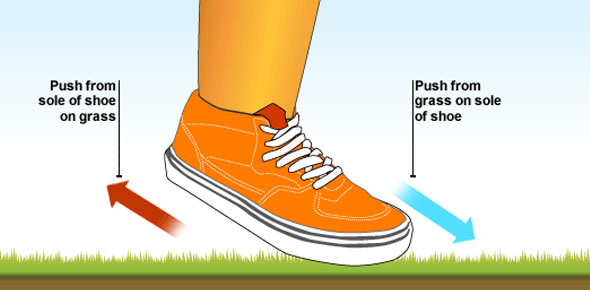# Chapter 2: Newton's First Law Of Motion, Inertia

10 Questions | Total Attempts: 562Settings• 1.
Whereas Aristotle relied on logic in explaining nature, Galileo relied on
• A.

Logic also

• B.

Patterns

• C.

Experiment

• D.

Mathematics

• 2.
The scientist to first introduce the concept of inertia was
• A.

Aristotle

• B.

Galileo

• C.

Newton

• D.

Copernicus

• 3.
Galileo's interpretation of motion differed from Aristotle's in that Galileo emphasized
• A.

The acceleration of free fall

• B.

Time rates

• C.

The role of distance in describing motion

• D.

All of these

• E.

None of these

• 4.
A sheet of paper can be withdrawn from under a container of milk without toppling it if the paper is jerked quickly. This best demonstrates that
• A.

The milk carton has no acceleration

• B.

There is an action-reaction pair of forces

• C.

Gravity tends to hold the milk carton secure

• D.

The milk carton has inertia

• E.

None of these

• 5.
An object in mechanical equilibrium is an object
• A.

At rest

• B.

Moving with constant velocity

• C.

Having no acceleration

• D.

All of these

• 6.
When you stand at rest on a pair of bathroom scales, the readings on the scales will always
• A.

• B.

• C.

• 7.
Hang from a pair of gym rings and the upward support forces of the rings will always
• A.

• B.

• C.

• 8.
A man weighing 800 N stands at rest on two bathroom scales so that his weight is distributed evenly over both scales. The reading on each scale is
• A.

200 N

• B.

400 N

• C.

800 N

• D.

None of these

• 9.
The force of friction on a sliding object is 10 N. The applied force needed to maintain a constant velocity is
• A.

More than 10 N

• B.

Less than 10 N

• C.

10 N

• 10.
A 300-kg bear grasping a vertical tree slides down at constant velocity. The friction force between the tree and the bear is
• A.

30 N

• B.

300 N

• C.

3000 N

• D.

More than 3000 N

Related TopicsBack to top